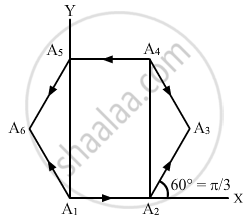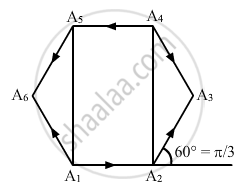Department of Pre-University Education, KarnatakaPUC Karnataka Science Class 11
Advertisement Remove all ads

# Let A1 A2 A3 A4 A5 A6 A1 Be a Regular Hexagon. Write The X-components of the Vectors Represented by the Six Sides Taken in Order. Use the Fact the Resultant of These Six Vectors is Zero, to Prove that - Physics

Answer in Brief

Let A1 A2 A3 A4 A5 A6 A1 be a regular hexagon. Write the x-components of the vectors represented by the six sides taken in order. Use the fact the resultant of these six vectors is zero, to prove that
cos 0 + cos π/3 + cos 2π/3 + cos 3π/3 + cos 4π/3 + cos 5π/3 = 0.
Use the known cosine values to verify the result.Advertisement Remove all ads

#### Solution

According to the polygon law of vector addition, the resultant of these six vectors is zero.
Here, a = b = c = d = e = f (magnitudes), as it is a regular hexagon. A regular polygon has all sides equal to each other.
So, $R_x = A \cos 0 + A \cos \frac{\pi}{3} + A \cos \frac{2\pi}{3} + A \cos \frac{3\pi}{3} + A \cos \frac{4\pi}{3} + A \cos \frac{5\pi}{3} = 0$

[As the resultant is zero, the x-component of resultant Rx is zero]

$\Rightarrow \cos 0 + \cos \frac{\pi}{3} + \cos \frac{2\pi}{3} + \cos\frac{3\pi}{3} + \cos \frac{4\pi}{3} + \cos \frac{5\pi}{5} = 0$Note: Similarly, it can be proven that

$\sin 0 + \sin \frac{\pi}{3} + \sin \frac{2\pi}{3} + \sin \frac{3\pi}{3} + \sin \frac{4\pi}{3} + \sin \frac{5\pi}{3} = 0$

Is there an error in this question or solution?
Advertisement Remove all ads

#### APPEARS IN

HC Verma Class 11, 12 Concepts of Physics 1
Chapter 2 Physics and Mathematics
Exercise | Q 12 | Page 29
Advertisement Remove all ads
Advertisement Remove all ads
Share
Notifications

View all notifications

Forgot password?